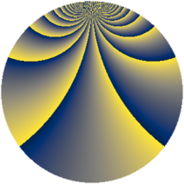# Properties

 Label 3549.2.dmLevel $3549$ Weight $2$ Character orbit 3549.dm Rep. character $\chi_{3549}(43,\cdot)$ Character field $\Q(\zeta_{78})$ Dimension $4416$ Sturm bound $970$

# Related objects

## Defining parameters

 Level: $$N$$ $$=$$ $$3549 = 3 \cdot 7 \cdot 13^{2}$$ Weight: $$k$$ $$=$$ $$2$$ Character orbit: $$[\chi]$$ $$=$$ 3549.dm (of order $$78$$ and degree $$24$$) Character conductor: $$\operatorname{cond}(\chi)$$ $$=$$ $$169$$ Character field: $$\Q(\zeta_{78})$$ Sturm bound: $$970$$

## Dimensions

The following table gives the dimensions of various subspaces of $$M_{2}(3549, [\chi])$$.

Total New Old
Modular forms 11760 4416 7344
Cusp forms 11568 4416 7152
Eisenstein series 192 0 192

## Trace form

 $$4416q - 188q^{4} + 184q^{9} + O(q^{10})$$ $$4416q - 188q^{4} + 184q^{9} + 12q^{11} + 8q^{13} + 12q^{15} + 196q^{16} - 8q^{17} - 24q^{20} - 76q^{22} + 4q^{23} + 352q^{25} - 40q^{26} + 4q^{29} - 8q^{30} - 104q^{31} - 12q^{33} - 260q^{34} - 188q^{36} - 12q^{37} + 56q^{38} + 8q^{39} + 40q^{40} + 12q^{41} + 4q^{42} - 24q^{43} + 12q^{45} + 12q^{46} + 16q^{48} - 184q^{49} - 60q^{50} + 24q^{51} + 256q^{52} + 4q^{53} + 160q^{55} - 64q^{58} + 84q^{59} + 208q^{60} + 4q^{61} + 240q^{62} + 448q^{64} + 64q^{65} - 192q^{66} - 64q^{67} + 8q^{68} - 4q^{69} - 104q^{74} + 368q^{76} - 16q^{77} + 236q^{78} - 112q^{79} - 144q^{80} + 184q^{81} + 280q^{82} - 76q^{85} - 224q^{87} + 956q^{88} - 96q^{89} + 8q^{91} + 56q^{92} - 80q^{94} + 216q^{95} + 60q^{97} + O(q^{100})$$

## Decomposition of $$S_{2}^{\mathrm{new}}(3549, [\chi])$$ into newform subspaces

The newforms in this space have not yet been added to the LMFDB.

## Decomposition of $$S_{2}^{\mathrm{old}}(3549, [\chi])$$ into lower level spaces

$$S_{2}^{\mathrm{old}}(3549, [\chi]) \cong$$ $$S_{2}^{\mathrm{new}}(169, [\chi])$$$$^{\oplus 4}$$$$\oplus$$$$S_{2}^{\mathrm{new}}(507, [\chi])$$$$^{\oplus 2}$$$$\oplus$$$$S_{2}^{\mathrm{new}}(1183, [\chi])$$$$^{\oplus 2}$$